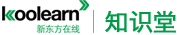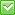<%/*%> <%*/%>热门：已解决问题
data sufficiency

If y is not equal 0 and y is not equal -1, which is greater, x/y or x/(y+1)?
(1) x is not equal 0
(2) x>y

stem(1) we just know x  (no sufficiency)
stem(2) we can not know the value of x and y  (no sufficiency)
together (1) and (2): we can know, which is greater, x/y or x/(y+1)

please tell me why I am wrong

Each person on a committee with 40 members voted for exactly one of 3

stem(1) we can not know the number of G and H  (no sufficiency)
stem(2) we can not know the number of F and G  (no sufficiency)
together (1) and (2) F=11   G=15     H=14   (sufficiency)最佳答案

1:请同学把详细题目发一下，谢谢。
The question is whether F received max votes of a total of 40.

Here if F were to receive the max votes then the total no. of votes would be < 31. But since the total no. of votes is 40, it goes to say that F did not recieve the max votes.